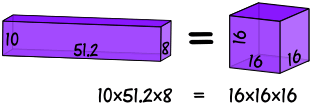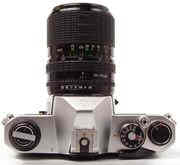# 几何平均数

### 例子：2 和 18 的几何平均数是多少？

• 先把数乘起来：2 × 18 = 36
• 然后（因为有两个数）开平方根：√36 = 6

2 和 18 的几何平均数 = √(2 × 18) = 6### 例子：10、51.2 和 8 的几何平均数是多少？

• 先把数相乘：10 × 51.2 × 8 = 4096
• 然后（因为有三个数）开立方根:3√4096 = 16

3√(10 × 51.2 × 8) = 16### 例子：1、3、9、27 和 81 的几何平均数是多少？

• 先把数相乘：1 × 3 × 9 × 27 × 81 = 59049
• 然后（因为有 5个数），开 5次方根：5√59049 = 9

1 × 3 × 9 × 27 × 81  =  9 × 9 × 9 × 9 × 9

## 定义

n个数：把所有数相乘，然后开 n 次方根（写成n

n√(a1 × a2 × …… × an)

## 应用### 例子：你想买照相机。

• 一个相机的变焦是 200，而品质评价是 8，
• 另一个的变焦是 250， 而品质评价是 6。.

• √(200 × 8) = 40
• √(250 × 6) = 38.7……